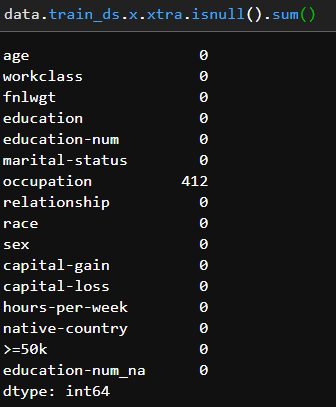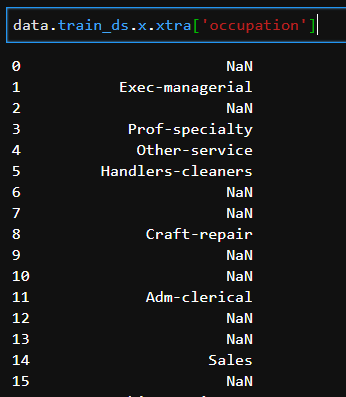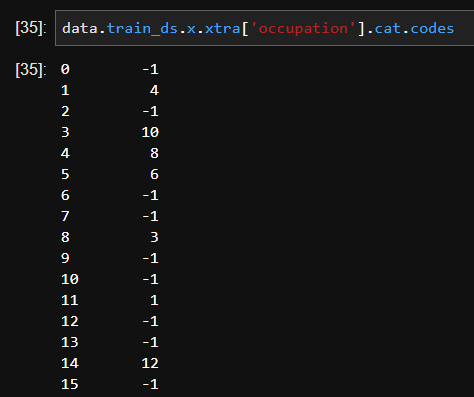# Error Getting Processed Dataframe back from TabularList

I created a gist here with a reproducible example.

My goal is simply to return the processed DataFrame back from the data object for further investigation. Unfortunately, after recent changes to the DataBlock API the method discussed here no longer works. I assumed the new .to_df() method replaced this functionality but it doesn’t appear to work. The gist includes several other methods of accessing the processed data without success.

Can anyone point me to the correct approach for returning the processed data back from the TabularList that gets created with the DataBlock API ?

I should add i’m using Ubuntu 18.04 and Fastai version 1.0.30

1 Like

You should be able to access the inner dataframe via `data.train_ds.x.xtra` (change train in valid/test).

So I actually try that in the gist but it returns the un-processed dataframe.

No it is processed (see the age column), you just see the categories instead of the codes, but if you ask .cat.codes on any of those categorical columns, you’ll see them.

Well it looks like it still contains missing values though:Actually, I’m still confused about the occupation column. It seems like in the df returned by xtra it still gives contains NaN’s and checking their codes confirms they’re still -1.It seems like FillMissing is working only on numeric columns and not categorical in this case ?

Actually looking at Categorify and FillMissing it doesn’t look like there is any handling of categorical missing values anymore? FillMissing seems dedicated to continuous only and no longer replaces cat.categories with codes and adds 1 so NaN’s = 0

``````    class Categorify(TabularProc):
"Transform the categorical variables to that type."
def apply_train(self, df:DataFrame):
self.categories = {}
for n in self.cat_names:
df.loc[:,n] = df.loc[:,n].astype('category').cat.as_ordered()
self.categories[n] = df[n].cat.categories

def apply_test(self, df:DataFrame):
for n in self.cat_names:
df.loc[:,n] = pd.Categorical(df[n], categories=self.categories[n], ordered=True)

@dataclass
class FillMissing(TabularProc):
"Fill the missing values in continuous columns."
fill_strategy:FillStrategy=FillStrategy.MEDIAN
fill_val:float=0.
def apply_train(self, df:DataFrame):
self.na_dict = {}
for name in self.cont_names:
if pd.isnull(df.loc[:,name]).sum():
df.loc[:,name+'_na'] = pd.isnull(df.loc[:,name])
if name+'_na' not in self.cat_names: self.cat_names.append(name+'_na')
if self.fill_strategy == FillStrategy.MEDIAN: filler = df.loc[:,name].median()
elif self.fill_strategy == FillStrategy.CONSTANT: filler = self.fill_val
else: filler = df.loc[:,name].dropna().value_counts().idxmax()
df.loc[:,name] = df.loc[:,name].fillna(filler)
self.na_dict[name] = filler``````

Here is a code snippet that would fix FillMissing:

``````class FillMissing(TabularProc):
"Fill the missing values in continuous columns."
fill_strategy:FillStrategy=FillStrategy.MEDIAN
fill_val:float=0.
def apply_train(self, df:DataFrame):
self.na_dict = {}
for name in self.cont_names:
if pd.isnull(df.loc[:,name]).sum():
df.loc[:,name+'_na'] = pd.isnull(df.loc[:,name])
if name+'_na' not in self.cat_names: self.cat_names.append(name+'_na')
if self.fill_strategy == FillStrategy.MEDIAN: filler = df.loc[:,name].median()
elif self.fill_strategy == FillStrategy.CONSTANT: filler = self.fill_val
else: filler = df.loc[:,name].dropna().value_counts().idxmax()
df.loc[:,name] = df.loc[:,name].fillna(filler)
self.na_dict[name] = filler
for name in self.cat_names:
if pd.isnull(df.loc[:,name]).sum():
df.loc[:,name+'_na'] = pd.isnull(df.loc[:,name])
if name+'_na' not in self.cat_names: self.cat_names.append(name+'_na')
df.loc[:,name] = df.loc[:,name].cat.codes+1``````

There is nothing to fix: the fastai library never replaced the NaNs in categorical variables. They were coded with -1 which is why we add +1 a little later to have codes that go from 0 (category nan) to len(classes).

oh ok, so I see that happens later on in TabularProcessor.process here:

``````if len(ds.cat_names) != 0:
ds.codes = np.stack([c.cat.codes.values for n,c in ds.xtra[ds.cat_names].items()], 1).astype(np.int64) + 1
``````

I guess that answers the original question then that data.train_ds.x.xtra isn’t finished processing yet.

My whole goal is to be able to access the same data that the NN is using so that I can perform model driven EDA using algorithms other than NN. To facilitate that on larger datasets it’d be nice to not have to duplicate the data in order to make final processing changes. I figured there must be a way to access the final data since this was a feature highlighted in this thread.

Final data is accessed by iterating through the dataloader: `for x,y in iter(data.train_dl)`